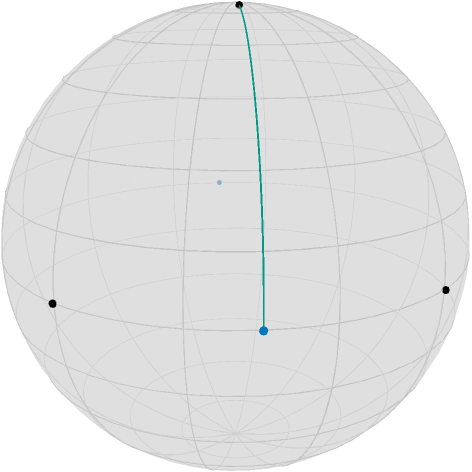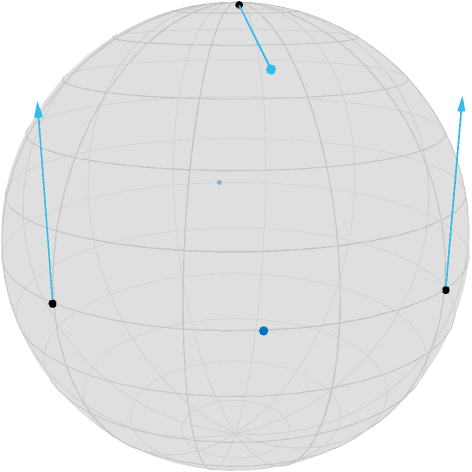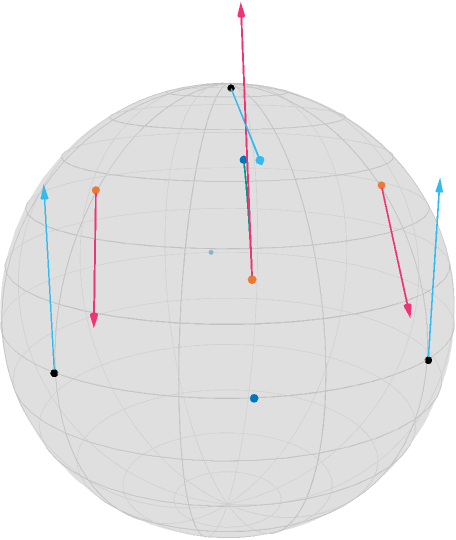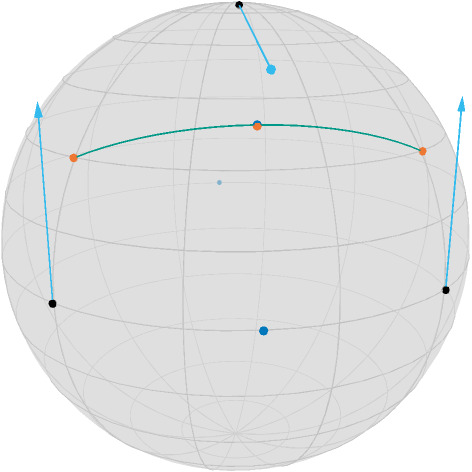# Illustration of the Gradient of a Second Order Difference

This example explains how to compute the gradient of the second order difference mid point model using adjoint_Jacobi_field

This example also illustrates the PowerManifold manifold as well as ArmijoLinesearch.

using Manopt, Manifolds, Colors, PlutoUI

We define some colors from Paul Tol

begin
black = RGBA{Float64}(colorant"#000000")
TolVibrantBlue = RGBA{Float64}(colorant"#0077BB")
TolVibrantOrange = RGBA{Float64}(colorant"#EE7733")
TolVibrantMagenta = RGBA{Float64}(colorant"#EE3377")
TolVibrantCyan = RGBA{Float64}(colorant"#33BBEE")
TolVibrantTeal = RGBA{Float64}(colorant"#009988")
end;
begin
T = [0:0.1:1.0...]
#render asy yes/no. If not, images included w/ markdown are assumed to be prerendered
render_asy = false
localpath = join(splitpath(@__FILE__)[1:(end - 1)], "/") # remove file to get files folder
image_prefix = localpath * "/second_order_difference"
@info image_prefix
end;

Assume we have two points $p,q$ on the equator of the Sphere $\mathcal M = \mathbb S^2$ and a point $r$ near the north pole

begin
M = Sphere(2)
p = [1.0, 0.0, 0.0]
q = [0.0, 1.0, 0.0]
c = mid_point(M, p, q)
r = shortest_geodesic(M, [0.0, 0.0, 1.0], c, 0.1)
end;

Now the second order absolute difference can be stated as [BacakBergmannSteidlWeinmann2016]

$$d_2(p_1,p:2,p_3) := \min_{c ∈ \mathcal C_{p_1,p_3}} d_{\mathcal M}(c,p_2),\qquad p_1,p_2,p_3∈\mathcal M,$$

where $\mathcal C_{p,q}$ is the set of all mid points $g(\frac{1}{2};p,q)$, between pand q, i.e. where $g$ is a (not necessarily minimizing) geodesic connecting both.

For illustration we further define the point opposite of the mid point c defined above

c2 = -c;

To illustrate the second order difference let‘s look at the geodesic connecting $r$ and the mid point $c$

geoPts_rc = shortest_geodesic(M, r, c, T);
render_asy && begin
asymptote_export_S2_signals(
image_prefix * "/SecondOrderData.asy";
curves=[geoPts_rc],
points=[[p, r, q], [c, c2]],
colors=Dict(:curves => [TolVibrantTeal], :points => [black, TolVibrantBlue]),
dot_size=3.5,
line_width=0.75,
camera_position=(1.2, 1.0, 0.5), #src
)
render_asymptote(image_prefix * "/SecondOrderData.asy"; render=2)
end;
PlutoUI.LocalResource(image_prefix * "/SecondOrderData.png")Figure. The three poins $p, q$, and $r$ (neart north pole, all black), which is connected by a geodesic (teal) to the mid point of the first two, $c$, (blue). The length of this geodesic is the cost of the second order total variation.

Since we moved $r$ 10% along the geodesic from the north pole to $c$, the distance to $c$ is $\frac{9\pi}{20}\approx 1.4137$, and this is also what the second order total variation cost, see costTV2, yields.

costTV2(M, (p, r, q))
1.413716694115407

But also its gradient can be easily computed since it is just a distance with respect to $r$ and a concatenation of a geodesic, where the start or end point is the argument, respectively, with a distance. Hence the adjoint differentials adjoint_differential_geodesic_startpoint and adjoint_differential_geodesic_endpoint can be employed. The gradient is also directly implemented, see grad_TV2. we obtain

(Xp, Xr, Xq) = grad_TV2(M, (p, r, q))
3-element Vector{Vector{Float64}}:
[-0.0, -4.9676995583751974e-18, -0.7071067811865475]
[-0.6984011233337103, -0.6984011233337102, 0.15643446504023084]
[4.9676995583751974e-18, 0.0, -0.7071067811865475]
render_asy && begin
asymptote_export_S2_signals(
points=[[p, r, q], [c, c2]],
tangent_vectors=[Tuple.([[p, -Xp], [r, -Xr], [q, -Xq]])],
colors=Dict(:tvectors => [TolVibrantCyan], :points => [black, TolVibrantBlue]),
dot_size=3.5,
line_width=0.75,
camera_position=(1.2, 1.0, 0.5),
)
end;
PlutoUI.LocalResource(image_prefix * "/SecondOrderGradient.png")Figure. The negative gradient of the second order difference cost indicates the movement of the three points in order to reduce their cost.

If we now perform a gradient step with constant step size 1, we obtain the three points

pn, rn, qn = exp.(Ref(M), [p, r, q], [-Xp, -Xr, -Xq])
3-element Vector{Vector{Float64}}:
[0.7602445970756302, 4.563951614149274e-18, 0.6496369390800624]
[0.6474502912317517, 0.6474502912317516, 0.4020152245473301]
[-4.563951614149274e-18, 0.7602445970756302, 0.6496369390800624]

as well we the new mid point

cn = mid_point(M, pn, qn)
3-element Vector{Float64}:
0.4508003528726723
0.4508003528726723
0.7704272085666162

Let‘s also again consider the geodesic connecting the new point $r_n$ and the new mid point $c_n$ as well as the gradient

begin
geoPts_rncn = shortest_geodesic(M, rn, cn, T)
(Xpn, Xrn, Xqn) = grad_TV2(M, (pn, rn, qn))
end;

The new configuration of the three points looks as follows

render_asy && begin
asymptote_export_S2_signals(
image_prefix * "/SecondOrderMin1.asy";
points=[[p, r, q], [c, c2, cn], [pn, rn, qn]],
curves=[geoPts_rncn],
tangent_vectors=[
Tuple.([[p, -Xp], [r, -Xr], [q, -Xq]]),
Tuple.([[pn, -Xpn], [rn, -Xrn], [qn, -Xqn]]),
],
colors=Dict(
:tvectors => [TolVibrantCyan, TolVibrantMagenta],
:points => [black, TolVibrantBlue, TolVibrantOrange],
:curves => [TolVibrantTeal],
),
dot_size=3.5,
line_width=0.75,
camera_position=(1.2, 1.0, 0.5),
)
render_asymptote(image_prefix * "/SecondOrderMin1.asy"; render=2)
end;
PlutoUI.LocalResource(image_prefix * "/SecondOrderMin1.png")Figure. The new situation of $p_n, q_n$, and $r_n$ (orange) and the miod point of the first two, $c_n$ (blue), which is again connected by a geodesic to $r_n$. Note that this geodesic is shorter, but also that $c$ and $r$ switched places. The new gradient (magenta) is only slightly reduced in magnitude.*

One can see, that this step slightly “overshoots”, i.e. $r$ is now even below $c$. and the cost function is still at

costTV2(M, (pn, rn, qn))
0.46579428818288593

But we can also search for the best step size using linesearch_backtrack on the PowerManifold manifold $\mathcal N = \mathcal M^3 = (\mathbb S^2)^3$

begin
x = [p, r, q]
N = PowerManifold(M, NestedPowerRepresentation(), 3)
s = linesearch_backtrack(N, x -> costTV2(N, x), x, grad_TV2(N, x), 1.0, 0.957, 0.999)
end
0.7362738857656108

and we obtain the new points

begin
pm, rm, qm = exp.(Ref(M), [p, r, q], s * [-Xp, -Xr, -Xq])
cm = mid_point(M, pm, qm)
geoPts_pmqm = shortest_geodesic(M, pm, qm, T)
end;

we obtain

render_asy && begin
asymptote_export_S2_signals(
image_prefix * "/SecondOrderMin2.asy";
points=[[p, r, q], [c, c2, cm], [pm, rm, qm]],
curves=[geoPts_pmqm],
tangent_vectors=[Tuple.([[p, -Xp], [r, -Xr], [q, -Xq]])],
colors=Dict(
:tvectors => [TolVibrantCyan],
:points => [black, TolVibrantBlue, TolVibrantOrange],
:curves => [TolVibrantTeal],
),
dot_size=3.5,
line_width=0.75,
camera_position=(1.2, 1.0, 0.5),
)
render_asymptote(image_prefix * "/SecondOrderMin2.asy"; render=2)
end;
PlutoUI.LocalResource(image_prefix * "/SecondOrderMin2.png")Figure. For the best step size found by line search, $r_m$ and $c_m$ nearly agree, i.e. $r_m$ lies on the geodesic between $p_m$ and $q_m$ as the geodesic drawn here indicates.

Here, the cost function yields

costTV2(M, (pm, rm, qm))
0.003907643132786784

which is much closer to zero, as one can also see, since the new center $c_m$ and $r_m$ are quite close.

## Literature

BacakBergmannSteidlWeinmann2016

Bačák, M., Bergmann, R., Steidl, G. and Weinmann, A.: A second order nonsmooth variational model for restoring manifold-valued images, SIAM Journal on Scientific Computations, Volume 38, Number 1, pp. A567–597, doi: 10.1137/15M101988X, arXiv: 1506.02409